$$\gdef\badDispatch#1{\textbf{\textcolor{e1432d}{#1}}} \gdef\noKatexForm#1{\badDispatch{#1}} \gdef\largeDot{\raisebox{0.06em}{\tiny∙}} \gdef\rarrbar{ {\raisebox{-0.05em}{→}\mkern{-0.13em}{\large\shortmid}}} \gdef\larrbar{ { {\large\shortmid}\mkern{-0.13em}{\raisebox{-0.05em}{←}}}} \gdef\suptrans{^\mathsf{T}} \gdef\supdagger{^\dagger} \gdef\rawsymbol#1{\operatorname{#1}} \gdef\colorsymbol#1#2{\textcolor{#1}{\rawsymbol{#2}}} \gdef\lsymbol#1{\colorsymbol{262626}{#1}} \gdef\msymbol#1{\colorsymbol{b50800}{#1}} \gdef\osymbol#1{\colorsymbol{00427f}{#1}} \gdef\lstring#1{\textcolor{666666}{\textrm{\textquotedblleft{\text{#1}}\textquotedblright}}} \gdef\boldForm#1{\textbf{#1}} \gdef\lrform#1{ {\textcolor{e1432d}{#1}}} \gdef\lgform#1{ {\textcolor{4ea82a}{#1}}} \gdef\lbform#1{ {\textcolor{3e81c3}{#1}}} \gdef\lrgform#1{ {\textcolor{ffc87d}{#1}}} \gdef\lgbform#1{ {\textcolor{96e2e3}{#1}}} \gdef\lrbform#1{ {\textcolor{f989b8}{#1}}} \gdef\rform#1{ {\textcolor{cb2b1a}{#1}}} \gdef\gform#1{ {\textcolor{399318}{#1}}} \gdef\bform#1{ {\textcolor{2b6fb0}{#1}}} \gdef\drform#1{ {\textcolor{9b0000}{#1}}} \gdef\dgform#1{ {\textcolor{006800}{#1}}} \gdef\dbform#1{ {\textcolor{004987}{#1}}} \gdef\drgform#1{ {\textcolor{ae5900}{#1}}} \gdef\dgbform#1{ {\textcolor{006567}{#1}}} \gdef\drbform#1{ {\textcolor{86004d}{#1}}} \gdef\rgform#1{ {\textcolor{dc841a}{#1}}} \gdef\gbform#1{ {\textcolor{47a5a7}{#1}}} \gdef\rbform#1{ {\textcolor{c74883}{#1}}} \gdef\pform#1{ {\textcolor{665996}{#1}}} \gdef\dpform#1{ {\textcolor{41326b}{#1}}} \gdef\waform#1{ {\textcolor{6b6b6b}{#1}}} \gdef\wbform#1{ {\textcolor{929292}{#1}}} \gdef\wcform#1{ {\textcolor{c5c5c5}{#1}}} \gdef\tick{\checkmark} \gdef\barToken{\mathbf{|}} \gdef\filledRectangleToken{▮} \gdef\emptyRectangleToken{▯} \gdef\filledSquareToken{■} \gdef\emptySquareToken{□} \gdef\filledToken{∙} \gdef\emptyToken{∘} \gdef\cardinalRewrite#1#2{\rewrite{#1}{#2}} \gdef\primed#1{ {#1}^{\prime}} \gdef\tinybullet{ {\tiny●}} \gdef\factsym#1{\mathord{#1}} \gdef\forwardFactor{\factsym{\uparrow}} \gdef\backwardFactor{\factsym{\downarrow}} \gdef\forwardBackwardFactor{\factsym{\updownarrow}} \gdef\forwardBackwardNeutralFactor{\factsym{\mathrlap{\downarrow}{\mathrlap{\uparrow}{\endash} } }} \gdef\neutralFactor{\factsym{\shornarrow}} \gdef\arrowhead{⏵} \gdef\inverseArrowhead{⏴} \gdef\rightArrowhead{⏵} \gdef\leftArrowhead{⏴} \gdef\upArrowhead{⏶} \gdef\downArrowhead{⏷} \gdef\de#1#2{ { {#1}\xundirectededge{}{#2}}} \gdef\ue#1#2{ { {#1}\xrightedge{}{#2}}} \gdef\shifttag#1{\raisebox{-1em}{#1}} \gdef\shifttag#1{#1} \gdef\tde#1#2#3{ {#1}\:\xrightedge{\shifttag{#3}}\;{#2}} \gdef\tue#1#2#3{ {#1}\;\xundirectededge{\shifttag{#3}}\;{#2}} \gdef\shiftunderset#1#2{\underset{\raisebox{0.15em}{\scriptsize #1}}{#2}} \gdef\mdtde#1#2#3#4{ {#1}\,\;\shiftunderset{#4}{\xrightedge{#3}}\;{#2}} \gdef\mtue#1#2#3#4{ {#1}\,\;\shiftunderset{#4}{\xundirectededge{#3}}\;\,{#2}} \gdef\mtde#1#2#3#4{ {#1}\,\;\operatornamewithlimits{\xrightedge{#3}}\limits_{#4} \;{#2}} \gdef\mapsfrom{\htmlClass{hreflect}{\mapsto}} \gdef\longmapsfrom{\htmlClass{hreflect}{\longmapsto}} \gdef\diffd{𝕕} \gdef\partialdof#1{\partial {#1}} \gdef\emptySet{\empty} \gdef\unknown{\wbform{\text{?}}} \gdef\notApplicable{\wbform{\text{---}}} \gdef\textAnd{\text{\,and\,}} \gdef\identicallyEqualSymbol{\equiv} \gdef\congruentSymbol{\equiv} \gdef\isomorphicSymbol{\simeq} \gdef\homeomorphicSymbol{\cong} \gdef\homotopicSymbol{\simeq} \gdef\approxEqualSymbol{\approx} \gdef\bijectiveSymbol{\approx} \gdef\defeq{\mathrel{≝}} \gdef\defEqualSymbol{\mathrel{≝}} \gdef\syntaxEqualSymbol{\mathrel{\textcolor{888888}{\equiv}}} \gdef\tailEqualSymbol{\underdot{=}} \gdef\headEqualSymbol{\dot{=}} \gdef\ruledelayed{:\to} \gdef\mathdollar{\text{\textdollar}} \gdef\hyphen{\text{-}} \gdef\endash{\text{--}} \gdef\emdash{\text{---}} \gdef\updownarrows{\uparrow\!\downarrow} \gdef\vthinspace{\mkern{1pt}} \gdef\dlq{\text{\textquotedblleft}} \gdef\drq{\text{\textquotedblright}} \gdef\dprime{ {\prime\prime}} \gdef\inverse#1{ { {#1}^{-1}}} \gdef\inverseSymbol{\inverse{□}} \gdef\groupDirectProduct#1{#1} \gdef\groupDirectProductSymbol{\times} \gdef\groupInverse{\inverse} \gdef\groupPower#1#2{ {#1}^{#2}} \gdef\groupCommutator#1#2{\left[{#1},{#2}\right]} \gdef\groupoidInverse{\inverse} \gdef\latticeBFS#1{\textrm{bfs}({#1})} \gdef\pathHomomorphism#1{#1} \gdef\groupoidFunction#1{#1} \gdef\groupoidHomomorphism#1{#1} \gdef\affineModifier#1{\overrightharpoon{#1}} \gdef\supercirc#1{#1^{\circ}} \gdef\supercircb#1{#1^{\bullet}} \gdef\blackCircleModifier#1{\supercircb{#1}} \gdef\whiteCircleModifier#1{\supercirc{#1}} \gdef\totalSpaceStyle#1{\rbform{#1}} \gdef\baseSpaceStyle#1{\bform{#1}} \gdef\fiberSpaceStyle#1{\rform{#1}} \gdef\totalSpaceElementStyle#1{\drbform{#1}} \gdef\baseSpaceElementStyle#1{\dbform{#1}} \gdef\fiberSpaceElementStyle#1{\drform{#1}} \gdef\bundleProjectionStyle#1{\dgform{#1}} \gdef\bundleGraphStyle#1{\rbform{#1}} \gdef\bundleSectionStyle#1{\drgform{#1}} \gdef\bundleFunctionStyle#1{\drbform{#1}} \gdef\graphFunctionStyle#1{\totalSpaceStyle{#1}} \gdef\projectionFunctionStyle#1{\totalSpaceStyle{#1}} \gdef\sectionFunctionStyle#1{\totalSpaceStyle{#1}} \gdef\functionGraph#1{G_{#1}} \gdef\toroidalModifier#1{\supercirc{#1}} \gdef\modulo#1{\supercirc{#1}} \gdef\dividesSymbol{\mathrel{|}} \gdef\groupFunction#1{#1} \gdef\groupHomomorphism#1{#1} \gdef\automorphisms{\operatorname{Aut}} \gdef\endomorphisms{\operatorname{End}} \gdef\transportMap#1{\transportMapSymbol_{#1}} \gdef\transportMapSymbol{\tau} \gdef\action#1{#1} \gdef\selfAction#1{\hat{#1}} \gdef\actionGroupoid#1{\utilde{#1}} \gdef\cardinalGroup#1{G^*({#1})} \gdef\signed#1{ {#1}^*} \gdef\transportAtlas#1{T_{#1}} \gdef\inverted#1{\underline{#1}} \gdef\mirror#1{\overline{#1}} \gdef\pathComposeSymbol{\mathbin{∷}} \gdef\pathCompose#1#2{ {#1}\pathComposeSymbol{#2}} \gdef\translateSymbol{\mathbin{\uparrow}} \gdef\backwardTranslateSymbol{\mathbin{\downarrow}} \gdef\pathTranslate#1#2{ {#1}\translateSymbol{#2}} \gdef\pathLeftTranslate#1{ { {#1}\translateSymbol}} \gdef\pathBackwardTranslate#1#2{ {#1}{\backwardTranslateSymbol}{#2}} \gdef\pathLeftBackwardTranslate#1{ {#1}{\backwardTranslateSymbol}} \gdef\compactCovariantDifference#1#2{\Delta_{#1}\,{#2}} \gdef\covariantDifference{ {#1}\,\Delta\,{#2}} \gdef\forwardDifference{\Delta^{+}} \gdef\backwardDifference{\Delta^{-}} \gdef\centralDifference{\Delta} \gdef\pathForwardDifference#1{\forwardDifference_{#1}} \gdef\pathBackwardDifference#1{\backwardDifference_{#1}} \gdef\pathCentralDifference#1{\centralDifference_{#1}} \gdef\pathHead#1{\pathHeadVector{#1}} \gdef\pathTail#1{\pathTailVector{#1}} \gdef\pathHeadVector#1{ {#1}^{\bullet}} \gdef\pathTailVector#1{ {#1}_{\bullet}} \gdef\pathReverse#1{ {#1}^{\dagger}} \gdef\pathIntegral#1#2{ {#1} \int {#2}} \gdef\pathIntegralSymbol{ {\int}} \gdef\pathDot#1#2{ {#1} \cdot {#2}} \gdef\pathDotSymbol{ {\cdot}} \gdef\compactBasis#1{\mathscr{B}} \gdef\length{\operatorname{len}} \gdef\signedLength{\operatorname{len^*}} \gdef\andFn{\operatorname{and}} \gdef\orFn{\operatorname{or}} \gdef\notFn{\operatorname{not}} \gdef\vertexList{\operatorname{vertices}} \gdef\vertexList{\operatorname{vertices}} \gdef\edgeList{\operatorname{edges}} \gdef\pathList{\operatorname{paths}} \gdef\cardinalList{\operatorname{cards}} \gdef\signedCardinalList{\operatorname{cards^*}} \gdef\wordOf{\operatorname{word}} \gdef\headVertex{\operatorname{head}} \gdef\tailVertex{\operatorname{tail}} \gdef\basis{\operatorname{basis}} \gdef\split{\operatorname{split}} \gdef\lcm{\operatorname{lcm}} \gdef\minimalContractionSets{\operatorname{MCSets}} \gdef\minimalContractions{\operatorname{MC}} \gdef\grade{\operatorname{grade}} \gdef\support{\operatorname{support}} \gdef\coefficient{\operatorname{coeff}} \gdef\domain{\operatorname{domain}} \gdef\codomain{\operatorname{codomain}} \gdef\modFunction{\operatorname{mod}} \gdef\clip{\operatorname{clip}} \gdef\sign{\operatorname{sign}} \gdef\step{\operatorname{step}} \gdef\projection{\operatorname{proj}} \gdef\lift{\operatorname{lift}} \gdef\identity{\operatorname{id}} \gdef\total{\operatorname{total}} \gdef\torus{\operatorname{torus}} \gdef\mobius{\operatorname{mobius}} \gdef\stateCompose{\operatorname{glue}} \gdef\infixStateComposeSymbol{\_} \gdef\stateDecompose{\operatorname{melt}} \gdef\stateJoin{\operatorname{conj}} \gdef\stateMeet{\operatorname{disj}} \gdef\stateExtent{\operatorname{extent}} \gdef\stateIntent{\operatorname{intent}} \gdef\infixStateJoinSymbol{\sqcup} \gdef\infixStateMeetSymbol{\sqcap} \gdef\isPrime#1{#1\textrm{ prime}} \gdef\blank{\_} \gdef\emptyWord{} \gdef\multiwordSymbol#1{\mathbf{#1}} \gdef\wordSymbol#1{\mathtt{#1}} \gdef\word#1{#1} \gdef\pathMap#1{#1} \gdef\function#1{#1} \gdef\imageModifier#1{ {#1}^{\rightarrow}} \gdef\preimageModifier#1{ {#1}^{\leftarrow}} \gdef\multiImageColorModifier#1{\msetCol{#1}} \gdef\multiImageModifier#1{ {#1}^{\Rightarrow}} \gdef\multiPreimageModifier#1{ {#1}^{\Leftarrow}} \gdef\functionComposition#1{#1} \gdef\functionCompositionSymbol{\mathbin{\small ∘}} \gdef\route#1#2#3{[{#1}\!:\!{#2}\!:\!{#3}]} \gdef\multiroute#1#2#3{[{#1}\!:\!{#2}\!:\!{#3}]} \gdef\pathWord#1#2#3{ {#1}\!:\!{#2}\!:\!{#3}} \gdef\parenPathWord#1#2#3{\left(\pathWord{#1}{#2}{#3}\right)} \gdef\nullPath{\bot} \gdef\nullElement{\bot} \gdef\path#1{#1} \gdef\vert#1{#1} \gdef\underdot#1{\underset{\raisebox{0.3em}{.}}{#1}} \gdef\headVertexSymbol{◨} \gdef\tailVertexSymbol{◧} \gdef\placeholderVertexSymbol{\mathrlap{◨}{◧}} \gdef\tvert#1{\underdot{#1}} \gdef\hvert#1{\dot{#1}} \gdef\edge#1{#1} \gdef\card#1{\mathtt{#1}} \gdef\path#1{#1} \gdef\quiver#1{#1} \gdef\bindingRuleSymbol{\to} \gdef\compactBindingRuleSymbol{:} \gdef\cayleyQuiverSymbol#1{\selfAction{#1}} \gdef\bindCayleyQuiver#1#2{\selfAction{#1}[#2]} \gdef\bindActionQuiver#1#2{#1[#2]} \gdef\bindSize#1#2{#1(#2)} \gdef\bindCardSize#1#2{#1[#2]} \gdef\bindCards#1#2{#1[#2]} \gdef\subSize#1#2{#1_{#2}} \gdef\gridQuiver#1{\textrm{Grid}^{#1}} \gdef\treeQuiver#1{\textrm{Tree}^{#1}} \gdef\bouquetQuiver#1{\textrm{Bq}^{#1}} \gdef\lineQuiver{\textrm{L}} \gdef\cycleQuiver{\textrm{C}} \gdef\squareQuiver{\textrm{Sq}} \gdef\cubicQuiver{\textrm{Cbc}} \gdef\triangularQuiver{\textrm{Tri}} \gdef\hexagonalQuiver{\textrm{Hex}} \gdef\rhombilleQuiver{\textrm{Rmb}} \gdef\limit#1#2{\lim_{#2}\,#1} \gdef\realVectorSpace#1{\mathbb{R}^{#1}} \gdef\complexVectorSpace#1{\mathbb{C}^{#1}} \gdef\matrixRing#1#2{M_{#2}(#1)} \gdef\groupoid#1{#1} \gdef\group#1{#1} \gdef\field#1{#1} \gdef\ring#1{#1} \gdef\indeterminate#1{#1} \gdef\semiring#1{#1} \gdef\sym#1{#1} \gdef\matrix#1{#1} \gdef\polynomial#1{#1} \gdef\setLetter{\mathcal{S}} \gdef\signedSetLetter{\mathcal{S^*}} \gdef\multisetLetter{\mathcal{M}} \gdef\signedMultisetLetter{\mathcal{M^*}} \gdef\multisetSemiringSymbol#1{#1} \gdef\multisetSemiring#1#2{\multisetLetter\left[#1, #2\right]} \gdef\signedMultisetRingSymbol#1{#1} \gdef\signedMultisetRing#1#2{\signedMultisetLetter\left[#1, #2\right]} \gdef\polynomialRing#1#2{#1[{#2}]} \gdef\routeSymbol#1{#1} \gdef\multirouteSymbol#1{\mathbf{#1}} \gdef\planSymbol#1{\mathbf{#1}} \gdef\ringElement#1{#1} \gdef\matrixPart#1#2#3{#1\llbracket{#2,#3}\rrbracket} \gdef\matrixRowPart#1{#1} \gdef\matrixColumnPart#1{#1} \gdef\subMatrixPart#1#2#3{#1_{#2,#3}} \gdef\matrixDotSymbol{\cdot} \gdef\matrixPlusSymbol{+} \gdef\wordGroup#1{\wordGroupSymbol_{#1}} \gdef\wordGroupSymbol{\Omega} \gdef\wordRing#1{\wordRingSymbol_{#1}} \gdef\wordRingSymbol{\Omega\!\degree} \gdef\linearCombinationCoefficient#1{ {\textcolor{888888}{#1}}} \gdef\plan#1{#1} \gdef\planRing#1{\planRingSymbol_{#1}} \gdef\planRingSymbol{\Phi} \gdef\basisPath#1#2{\mathbf{#1}_{#2}} \gdef\basisPathWeight#1#2{ {#1}_{#2}} \gdef\unitSymbol{\mathbf{e}} \gdef\unitVertexField{\unitSymbol_1} \gdef\forwardSymbol{f} \gdef\backwardSymbol{b} \gdef\symmetricSymbol{s} \gdef\antisymmetricSymbol{a} \gdef\wordVector#1#2{\unitSymbol_{#1}^{#2}} \gdef\gradOf#1{\grad\,{#1}} \gdef\grad{\nabla} \gdef\divOf#1{\div\,{#1}} \gdef\div{\dot{\nabla}} \gdef\laplacianOf#1{\laplacian\,{#1}} \gdef\laplacian{\ddot{\nabla}} \gdef\suchThat#1#2{ {#1}\,\big|\,{#2}} \gdef\chart#1{\chartSymbol_{#1}} \gdef\chartSymbol{C} \gdef\graphRegionIntersectionSymbol{\cap} \gdef\graphRegionUnionSymbol{\cup} \gdef\pathIso{\simeq} \gdef\factorial#1{#1!} \gdef\power#1#2{ {#1}^{#2}} \gdef\repeatedPower#1#2{ {#1}^{#2}} \gdef\contractionLattice#1{\operatorname{Con}(#1)} \gdef\contractedRelation#1{\sim_{#1}} \gdef\isContracted#1#2{ {#1} \sim {#2}} \gdef\isContractedIn#1#2#3{ {#1} \sim_{#3} {#2}} \gdef\isNotContracted#1#2{ {#1} \not \sim {#2}} \gdef\isNotContractedIn#1#2#3{ {#1} \not \sim_{#3} {#2}} \gdef\contractionSum#1{#1} \gdef\contractionSumSymbol{\sqcup} \gdef\contractionProduct#1{#1} \gdef\contractionProductSymbol{ {\cdot}} \gdef\graph#1{#1} \gdef\graphHomomorphism#1{#1} \gdef\coversSymbol{\sqsupseteq} \gdef\coveredBySymbol{\sqsubseteq} \gdef\strictlyCoversSymbol{\sqsupset} \gdef\strictlyCoveredBySymbol{\sqsubset} \gdef\graphCovering#1#2#3{ {#2} \sqsupseteq_{#1} {#3}} \gdef\quiverCovering#1#2#3{ {#2} \sqsupseteq^{#1} {#3}} \gdef\powerSetSymbol{\mathcal{P}} \gdef\powerSet#1{\powerSetSymbol({#1})} \gdef\existsForm#1#2{\exists\,{#1}\,:\,{#2}} \gdef\forAllForm#1#2{\forall\,{#1}\,:\,{#2}} \gdef\notted#1{\notSymbol {#1}} \gdef\andSymbol{\land} \gdef\orSymbol{\lor} \gdef\notSymbol{\lnot} \gdef\latticeSymbol#1{#1} \gdef\meetSemilatticeSymbol#1{#1} \gdef\joinSemilatticeSymbol#1{#1} \gdef\posetSymbol#1{#1} \gdef\latticeElementSymbol#1{#1} \gdef\meetSemilatticeElementSymbol#1{#1} \gdef\joinSemilatticeElementSymbol#1{#1} \gdef\posetElementSymbol#1{#1} \gdef\latticeMeetSymbol{\wedge} \gdef\latticeJoinSymbol{\vee} \gdef\latticeTop{\top} \gdef\latticeBottom{\bot} \gdef\semilatticeMeetSymbol{\wedge} \gdef\semilatticeJoinSymbol{\vee} \gdef\semilatticeTop{\top} \gdef\semilatticeBottom{\bot} \gdef\semilatticeSemimeetSymbol{\wedge} \gdef\semilatticeSemijoinSymbol{\vee} \gdef\latticeGreaterSymbol{>} \gdef\latticeGreaterEqualSymbol{\ge} \gdef\latticeLessSymbol{<} \gdef\latticeLessEqualSymbol{\le} \gdef\posetGreaterSymbol{>} \gdef\posetGreaterEqualSymbol{\ge} \gdef\posetLessSymbol{<} \gdef\posetLessEqualSymbol{\le} \gdef\posetCoversSymbol{⋗} \gdef\posetCoveredBySymbol{⋖} \gdef\grpname#1{\operatorname{\mathsf{#1}}} \gdef\mkg#1#2#3{\grpname{#1}({#2},{#3})} \gdef\mka#1#2#3{\mathfrak{#1}({#2},{#3})} \gdef\generalLinearAlgebra#1#2{\mka{gl}{#1}{#2}} \gdef\generalLinearGroup#1#2{\mkg{GL}{#1}{#2}} \gdef\specialLinearAlgebra#1#2{\mka{sl}{#1}{#2}} \gdef\specialLinearGroup#1#2{\mkg{SL}{#1}{#2}} \gdef\projectiveGeneralLinearAlgebra#1#2{\mka{pgl}{#1}{#2}} \gdef\projectiveGeneralLinearGroup#1#2{\mkg{PGL}{#1}{#2}} \gdef\projectiveSpecialLinearAlgebra#1#2{\mka{psl}{#1}{#2}} \gdef\projectiveSpecialLinearGroup#1#2{\mkg{PSL}{#1}{#2}} \gdef\orthogonalAlgebra#1#2{\mka{o}{#1}{#2}} \gdef\orthogonalGroup#1#2{\mkg{O}{#1}{#2}} \gdef\specialOrthogonalAlgebra#1#2{\mka{so}{#1}{#2}} \gdef\specialOrthogonalGroup#1#2{\mkg{SO}{#1}{#2}} \gdef\unitaryAlgebra#1{\mka{u}{#1}{#2}} \gdef\unitaryGroup#1{\mkg{U}{#1}{#2}} \gdef\specialUnitaryAlgebra#1#2{\mka{su}{#1}{#2}} \gdef\specialUnitaryGroup#1#2{\mkg{su}{#1}{#2}} \gdef\spinAlgebra#1#2{\mka{spin}{#1}{#2}} \gdef\spinGroup#1#2{\mkg{Spin}{#1}{#2}} \gdef\pinAlgebra#1#2{\mka{pin}{#1}{#2}} \gdef\pinGroup#1#2{\mkg{Pin}{#1}{#2}} \gdef\symmetricGroup#1{\grpname{Sym}({#1})} \gdef\presentation#1{#1} \gdef\groupPresentation#1#2{\left\langle\,{#1}\,\,\middle|\mathstrut\,\,{#2}\,\right\rangle} \gdef\groupRelationIso{=} \gdef\groupGenerator#1{#1} \gdef\groupRelator#1{#1} \gdef\groupElement#1{#1} \gdef\identityElement#1{#1} \gdef\groupoidElement#1{#1} \gdef\groupIdentity#1{#1} \gdef\groupoidIdentity#1{#1} \gdef\ringIdentity#1{#1} \gdef\iconstruct#1#2{ {#1}\,\,\middle|{\large\mathstrut}\,\,{#2}} \gdef\setConstructor#1#2{\left\{\,\iconstruct{#1}{#2}\,\right\}} \gdef\multisetConstructor#1#2{\left\{\mkern{-2.3pt}\left|\,\,\iconstruct{#1}{#2}\,\right|\mkern{-2.3pt}\right\}} \gdef\cardinalityConstructor#1#2{\left|\,\iconstruct{#1}{#2}\,\right|} \gdef\setToMultiset#1{ {#1}^\uparrow} \gdef\multisetToSet#1{ {#1}^\downarrow} \gdef\subsets#1{\setLetter({#1})} \gdef\signedSubsets#1{\signedSetLetter({#1})} \gdef\multisets#1{\multisetLetter({#1})} \gdef\signedMultisets#1{\signedMultisetLetter({#1})} \gdef\circleSpaceSymbol{S} \gdef\topologicalSpace#1{#1} \gdef\bundleSection#1{#1} \gdef\bundleProjection#1{#1} \gdef\setSymbol#1{#1} \gdef\signedSetSymbol#1{#1} \gdef\multisetSymbol#1{#1} \gdef\signedMultisetSymbol#1{#1} \gdef\setElementSymbol#1{#1} \gdef\signedSetElementSymbol#1{#1} \gdef\multisetElementSymbol#1{#1} \gdef\signedMultisetElementSymbol#1{#1} \gdef\negated#1{\bar{#1}} \gdef\positiveSignedPart#1{ {#1}^+} \gdef\negativeSignedPart#1{ {#1}^-} \gdef\multisetMultiplicitySymbol{\,\raisebox{.1em}{\small\#}\mkern{.1em}\,} \gdef\signedMultisetMultiplicitySymbol{\,\raisebox{.1em}{\small\#}\mkern{.1em}\,} \gdef\boundMultiplicityFunction#1{ {#1}^{\sharp}} \gdef\boundSignedMultiplicityFunction#1{\operatorname{ {#1}^{\sharp}}} \gdef\constructor#1#2{\left.{#1}\,\,\middle|\mathstrut\,\,{#2}\right.} \gdef\elemOf#1#2{ { {#1} \in {#2} }} \gdef\notElemOf#1#2{ { {#1} \notin {#2} }} \gdef\edgeOf#1#2{ { {#1} {\in}_E {#2} }} \gdef\vertOf#1#2{ { {#1} {\in}_V {#2} }} \gdef\pathOf#1#2{ { {#1} {\in}_P {#2} }} \gdef\pathType#1{\operatorName{path}{#1}} \gdef\vertexType#1{\operatorName{vertex}{#1}} \gdef\edgeType#1{\operatorName{edge}{#1}} \gdef\multisetType#1{\operatorName{mset}{#1}} \gdef\signedMultisetType#1{\operatorName{mset^*}{#1}} \gdef\unitInterval{\mathbb{I}} \gdef\fromTo#1{#1} \gdef\fromToSymbol{\mapsto} \gdef\vertexCountOf#1{|{#1}|} \gdef\vertices#1{ V_{#1} } \gdef\edges#1{ E_{#1} } \gdef\vertexField#1{#1} \gdef\edgeField#1{#1} \gdef\pathVector#1{\mathbf{#1}} \gdef\pathVectorSpace#1{\mathscr{#1}} \gdef\baseField#1{#1} \gdef\finiteField#1{\mathbb{F}_{#1}} \gdef\functionSpace#1#2{#2^{#1}} \gdef\finiteTotalFunctionSpace#1#2{#2^{\sub #1}} \gdef\pathGroupoid#1{ { \Gamma_{#1} }} \gdef\forwardPathQuiver#1#2{ {\overrightharpoon{#1}_{#2}}} \gdef\backwardPathQuiver#1#2{ {\overrightharpoon{#1}^{#2}}} \gdef\pathQuiver#1{ {\overrightharpoon{#1}}} \gdef\mto#1#2{ {#1}\mapsto{#2}} \gdef\mtoSymbol{\mapsto} \gdef\groupWordRewriting#1{\langle{#1}\rangle} \gdef\rewrite#1#2{ {#1}\mapsto{#2}} \gdef\rewritingRule#1#2{ {#1}\mapsto{#2}} \gdef\rewritingSystem#1{\mathcal{#1}} \gdef\multiwayBFS#1{\textrm{bfs}({#1})} \gdef\rewritingStateBinding#1#2{ {\left.{#1}\!\mid\!{#2}\right.}} \gdef\rewritingRuleBinding#1#2{#1{\left[\,{#2}\,\right]}} \gdef\namedSystem#1{\mathtt{#1}} \gdef\genericRewritingSystem{\namedSystem{Sys}} \gdef\stringRewritingSystem{\namedSystem{Str}} \gdef\circularStringRewritingSystem{\namedSystem{CStr}} \gdef\turingMachineRewritingSystem{\namedSystem{TM}} \gdef\cellularAutomatonRewritingSystem{\namedSystem{CA}} \gdef\graphRewritingSystem{\namedSystem{Gr}} \gdef\hypergraphRewritingSystem{\namedSystem{HGr}} \gdef\petriNetRewritingSystem{\namedSystem{Petri}} \gdef\localStates#1{\localStatesSymbol^#1} \gdef\regionalStates#1{\regionalStatesSymbol^#1} \gdef\globalStates#1{\globalStatesSymbol^#1} \gdef\keySubStates#1{\keySubStatesSymbol^#1} \gdef\valueSubStates#1{\valueSubStatesSymbol^#1} \gdef\localState#1#2{#2_{#1}} \gdef\regionalState#1{#1} \gdef\globalState#1{#1} \gdef\keySubState#1{#1} \gdef\valueSubState#1{#1} \gdef\lhsState#1{#1_{L}} \gdef\rhsState#1{#1_{R}} \gdef\rewriteLHSRegionalState#1{#1_{L}} \gdef\rewriteRHSRegionalState#1{#1_{R}} \gdef\regionalStateForm#1{\waform{(}#1\waform{)}} \gdef\invalidRegionalState{\rform{\times}} \gdef\emptyRegionalState{\regionalStateForm{}} \gdef\regionalSubstateSymbol{\sqsubseteq} \gdef\regionalSuperstateSymbol{\sqsupseteq} \gdef\comparableRegionalStatesSymbol{\mathbin{\square}} \gdef\incomparableRegionalStatesSymbol{\mathbin{\boxtimes}} \gdef\namedStateSet#1{\mathbf #1} \gdef\localStatesSymbol{\namedStateSet L} \gdef\regionalStatesSymbol{\namedStateSet R} \gdef\globalStatesSymbol{\namedStateSet G} \gdef\keySubStatesSymbol{\namedStateSet L_K} \gdef\valueSubStatesSymbol{\namedStateSet L_V} \gdef\localStateSymbol#1{#1} \gdef\regionalStateSymbol#1{#1} \gdef\globalStateSymbol#1{#1} \gdef\keySubStateSymbol#1{#1} \gdef\valueSubStateSymbol#1{#1} \gdef\infixComposeLocalStatesSymbol{\_} \gdef\composeLocalStatesSymbol{\operatorname{glue}} \gdef\composeLocalStatesForm#1{\composeLocalStatesSymbol(#1)} \gdef\ncard#1{\card{\inverted{#1}}} \gdef\mcard#1{\card{\mirror{#1}}} \gdef\nmcard#1{\card{\inverted{\mirror{#1}}}} \gdef\assocArray#1{\left\langle {#1} \right\rangle} \gdef\openMultiset{\lBrace} \gdef\closeMultiset{\rBrace} \gdef\set#1{\{ {#1} \}} \gdef\signedSet#1{\{ {#1} \}} \gdef\list#1{\{ {#1} \}} \gdef\multiset#1{\openMultiset {#1} \closeMultiset} \gdef\signedMultiset#1{\openMultiset {#1} \closeMultiset} \gdef\styledSet#1#2{#1\{ {#1} #1\}} \gdef\styledList#1#2{#1\{ {#1} #1\}} \gdef\styledMultiset#1#2{#1\openMultiset {#2} #1\closeMultiset} \gdef\styledSignedMultiset#1#2{\openMultiset {#2} \closeMultiset} \gdef\permutationCycle#1{#1} \gdef\permutationCycleSymbol{\to} \gdef\permutationSet#1{#1} \gdef\permutationSetSymbol{;} \gdef\transposition#1#2{ {#1} \leftrightarrow {#2}} \gdef\tuple#1{( {#1} )} \gdef\concat#1{ {#1} } \gdef\paren#1{\left( {#1} \right)} \gdef\ceiling#1{\lceil{#1}\rceil} \gdef\floor#1{\lfloor{#1}\rfloor} \gdef\translationVector#1{\left[{#1}\right]_{\textrm{T}}} \gdef\quotient#1#2{ {#1} / {#2}} \gdef\compactQuotient#1#2#3{ {#1}\;{\upharpoonright_{#2}}\;{#3}} \gdef\multilineQuotient#1#2{\frac{#1}{#2}} \gdef\idElem#1{e_{#1}} \gdef\minus#1{-{#1}} \gdef\elem{\ \in\ } \gdef\vsp{\mkern{0.4pt}} \gdef\iGmult{\vsp} \gdef\gmult{\vsp\ast\vsp} \gdef\Gmult{\vsp\ast\vsp} \gdef\gdot{\vsp\cdot\vsp} \gdef\gDot{\vsp\mathbin{\largeDot}\vsp} \gdef\mdot{\vsp\cdot\vsp} \gdef\smallblackcirc{\vsp\raisebox{0.15em}{\tiny∙}\vsp} \gdef\smallwhitecirc{\vsp\raisebox{0.15em}{\tiny∘}\vsp} \gdef\sgdot{\mathbin{\smallwhitecirc}} \gdef\srdot{\mathbin{\smallblackcirc}} \gdef\srplus{+} \gdef\ellipsis{\,\wbform{...}\,} \gdef\verticalEllipsis{\vdots} \gdef\appliedRelation#1{#1} \gdef\setUnionSymbol{\cup} \gdef\setIntersectionSymbol{\cap} \gdef\setRelativeComplementSymbol{-} \gdef\msetCol{\textcolor{bb4444}} \gdef\repeatedMultiset#1#2{#1\,#2} \gdef\msrdot{\mathbin{\smallblackcirc}} \gdef\msrplus{+} \gdef\smrdot{\mathbin{\smallblackcirc}} \gdef\smrplus{+} \gdef\dotminus{\mathbin{\dot{-}}} \gdef\dotcap{\mathbin{\dot{\cap}}} \gdef\dotcup{\mathbin{\dot{\cup}}} \gdef\multisetUnionSymbol{\dotcup} \gdef\multisetIntersectionSymbol{\dotcap} \gdef\multisetRelativeComplementSymbol{\dotminus} \gdef\multisetSumSymbol{\dotplus} \gdef\cartesianProductSymbol{\times} \gdef\functionType#1#2{ {#1} \to {#2}} \gdef\functionSignature#1#2#3{ { {#1} : {#2} \to {#3}}} \gdef\partialFunctionSignature#1#2#3{ { {#1} : {#2} \rightharpoonup {#3}}} \gdef\poly#1{#1} \gdef\quiverProdPoly#1{#1} \gdef\quiverProdPower#1#2{#1^{#2}} \gdef\quiverProdTimes#1{#1} \gdef\parenLabeled#1#2{#1\ ({#2})} \gdef\parenRepeated#1#2{\parenLabeled{#1}{ {#2}\text{ times}}} \gdef\underLabeled#1#2{\underbrace{#1}_{#2}} \gdef\underRepeated#1#2{\underbrace{#1}_{#2\text{ times}}} \gdef\overLabeled#1#2{\overbrace{#1}^{#2}} \gdef\overRepeated#1#2{\overbrace{#1}^{#2\text{ times}}} \gdef\modLabeled#1#2{ {#1 }\textrm{ mod }{#2}} \gdef\freeGroup#1{F_{#1}} \gdef\cyclicGroup#1{\mathbb{Z}_{#1}} \gdef\componentSuperQuiverOfSymbol{\succ} \gdef\setCardinality#1{|{#1}|} \gdef\multisetCardinality#1{|{#1}|} \gdef\dependentQuiverProductSymbol{\mathbin{\times}} \gdef\rightIndependentQuiverProductSymbol{\mathbin{⋊}} \gdef\leftIndependentQuiverProductSymbol{\mathbin{⋉}} \gdef\rightStrongQuiverProductSymbol{\mathbin{⧒}} \gdef\leftStrongQuiverProductSymbol{\mathbin{⧑}} \gdef\rightFiberQuiverProductSymbol{\mathbin{⧕}} \gdef\leftFiberQuiverProductSymbol{\mathbin{⧔}} \gdef\lockedQuiverProductSymbol{\mathbin{\searrow}} \gdef\rightFreeQuiverProductSymbol{\mathbin{\smallerthan}} \gdef\leftFreeQuiverProductSymbol{\mathbin{\largerthan}} \gdef\strongIndependentQuiverProductSymbol{\mathbin{⨝}} \gdef\cartesianQuiverProductSymbol{\mathbin{□}} \gdef\strongQuiverProductSymbol{\mathbin{⊠}} \gdef\graphUnionSymbol{\sqcup} \gdef\graphProductSymbol{\times} \gdef\inlineProdSymbol{|} \gdef\serialCardSymbol{ {:}} \gdef\parallelCardSymbol{ {\mid}} \gdef\cardinalSequenceSymbol{ {:}} \gdef\cardinalProduct#1{(#1)} \gdef\vertexProduct#1{(#1)} \gdef\edgeProduct#1{(#1)} \gdef\cardinalProductSymbol{\inlineProdSymbol} \gdef\vertexProductSymbol{\inlineProdSymbol} \gdef\edgeProductSymbol{\inlineProdSymbol} \gdef\verticalVertexProduct#1#2{\cfrac{#1}{#2}} \gdef\verticalCardinalProduct#1#2{\cfrac{#1}{#2}} \gdef\indexSum#1#2#3{ {\sum_{#2}^{#3} #1}} \gdef\indexProd#1#2#3{ {\prod_{#2}^{#3} #1}} \gdef\indexMax#1#2#3{ {\max_{#2}^{#3} #1}} \gdef\indexMin#1#2#3{ {\min_{#2}^{#3} #1}} \gdef\indexUnion#1#2#3{ {\bigcup_{#2}^{#3} #1}} \gdef\indexIntersection#1#2#3{ {\bigcap_{#2}^{#3} #1}} \gdef\indexGraphUnion#1#2#3{ {\bigcup_{#2}^{#3} #1}} \gdef\indexGraphDisjointUnion#1#2#3{ {\bigcup_{#2}^{#3} #1}} \gdef\styledIndexSum#1#2#3#4{ {#1\sum_{#3}^{#4} #2}} \gdef\styledIndexProd#1#2#3#4{ {#1\prod_{#3}^{#4} #2}} \gdef\styledIndexMax#1#2#3#4{ {#1\max_{#3}^{#4} #2}} \gdef\styledIndexMin#1#2#3#4{ {#1\min_{#3}^{#4} #2}} \gdef\indexSumSymbol{\sum} \gdef\indexProdSymbol{\prod} \gdef\indexMaxSymbol{\max} \gdef\indexMinSymbol{\min} \gdef\openInterval#1#2{(#1,#2)} \gdef\closedInterval#1#2{[#1,#2]} \gdef\openClosedInterval#1#2{(#1,#2]} \gdef\closedOpenInterval#1#2{[#1,#2)} \gdef\oneTo#1{1..{#1}} \gdef\zeroTo#1{0..{#1}} \gdef\qstring#1{\mathtt{"}{#1}\mathtt{"}} \gdef\wstring#1{\textcolor{6b6b6b}{#1}} \gdef\qchar#1{\mathtt{'}{#1}\mathtt{'}} \gdef\lstr#1{\mathtt{#1}} \gdef\lchar#1{\mathtt{#1}} \gdef\string#1{ {#1}} \gdef\character#1{ {#1}} \gdef\homomorphismMapping#1{\assocArray{#1}} \gdef\starModifier#1{ {#1}^*} \gdef\translationPresentation#1{\textrm{Z}_{#1}} \gdef\starTranslationPresentation#1{\textrm{Z}^*_{#1}} \gdef\translationPathValuation#1{\mathcal{\overrightharpoon Z}_{#1}} \gdef\starTranslationPathValuation#1{\overrightharpoon{\mathcal{Z}^*_{#1}}} \gdef\translationWordHomomorphism#1{\mathcal{Z}_{#1}} \gdef\starTranslationWordHomomorphism#1{\mathcal{Z}^*_{#1}} \gdef\translationCardinalValuation#1{\textrm{T}_{#1}} \gdef\starTranslationCardinalValuation#1{\textrm{T}^*_{#1}}$$
Path calculus

# Path calculus

## Introduction

This section will introduce path calculus. Path calculus is the simple formalism that extends the so-called calculus of finite differences to the setting of functions defined on the vertices of quivers. In the subsequent section, we will explain how to generalize path calculus into the realm of path algebra, where finite differences become linear operators on a vector space of paths. But for now, the constructions will be elementary and straightforward.

How do we set up calculus on cardinal quivers? Before beginning, it is obvious that we should expect something that looks more like the calculus of finite differences than the more familiar infinitesimal calculus employed for continuous functions. Philosophically, the individual edges of a lattice quiver are the equivalent of infinitesimals from a suitably “zoomed out” perspective.

All the following examples will be hosted on a relatively simple lattice quivers. We’ll start with the one-dimensional line lattice, and move later to the square and triangular lattices to illustrate the generalization to multiple cardinals and hence dimensions.

## One dimensional lattices

To start, our base lattice will be line lattice, written $$\quiver{L}$$, which has the attractive pedagogical property that it will act like the discrete equivalent of the real number line on which continuous one-dimensional functions are usually defined.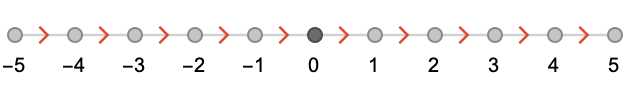We’ve labeled the vertices with integers, with 0 for the origin vertex. We’ll refer to the vertices of $$\quiver{L}$$ as $$\vertices{\quiver{L}} = \vertexList(\quiver{L})$$.

## Vertex fields

A vertex field on $$\quiver{L}$$ is a function $$\functionSignature{\function{\vertexField{f}}}{\vertices{\quiver{L}}}{\baseField{K}}$$, where $$\baseField{K}$$ is a field (or commutative ring). We write the space of all vertex fields $$\functionSpace{\vertices{}}{\baseField{K}}$$.

The word field here is serving two different roles, of course: the field in “vertex field” is analogous to a scalar field in physics: a single value at each point in space, whereas the field in “$$\baseField{K}$$ is a field refers to a field of scalar values in the sense of algebraic ring with inverses. Of course, vertex fields form a field in their own right under pointwise multiplication, addition, and inversion, so the pun is in some sense accurate.

We’ll initially consider only finite fields $$\baseField{K} = \finiteField{p}$$, which will allow us to visualize vertex fields as colorings of vertices. For $$\sym{p}=2$$ we’ll use white for 0 and black for 1, and for $$\sym{p}=3$$ we’ll use white for 0, red for 1, and blue for −1. For $$\sym{p}>3$$, the cyclic property of these finite fields will be represented with colors that cycle through the visible spectrum.

Here are two vertex fields $$\vertexField{u}$$ and $$\vertexField{v}$$ we’ll use to illustrate various operations:We can form sums and products of these vertex fields, defined vertex-wise: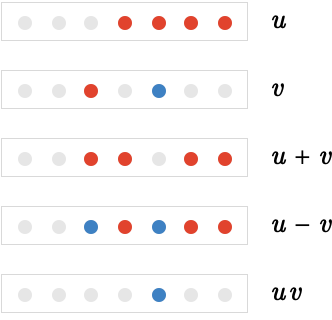## Translation operator

We now introduce the translation operator $$\pathTranslate{}{\vertexField{u}}$$, which translates the vertex field $$\vertexField{u}$$ along the cardinal $$\card{x}$$. Later we’ll define this operation for quivers with more than one cardinal, and generalize it to allow translation along arbitrary paths.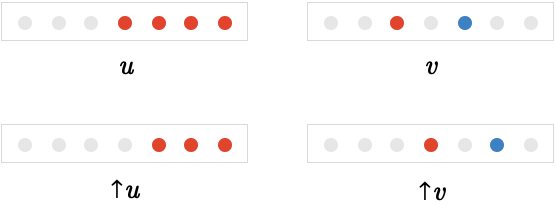Notice that the vertex fields $$\vertexField{u}$$ and $$\vertexField{v}$$ have been “shifted over” to the right. Similarly we can define the backward translation operator $$\pathBackwardTranslate{}{\vertexField{u}}$$: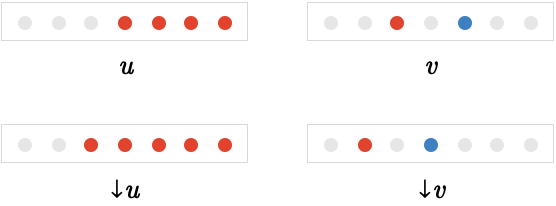## Finite differences

We can use these translation operators to define the forward finite difference of a vertex field $$\vertexField{u}$$, written $$\forwardDifference{}\,\vertexField{u}$$.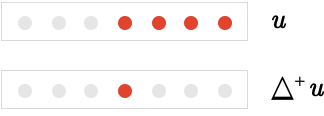The operation $$\forwardDifference{}\,\vertexField{u}$$ is defined to be $$\forwardDifference{}\,\vertexField{u} = \vertexField{u} - \pathTranslate{}{\vertexField{u}}$$, and can be thought of as comparing the translated and untranslated versions of $$\vertexField{u}$$: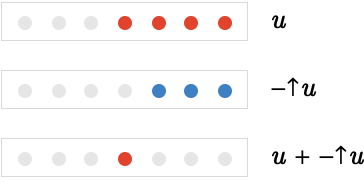The single red vertex in the center indicates that the vertex field $$\vertexField{u} = \list{\ellipsis ,0,0,1,1,1,\ellipsis }$$ has a “step change”, yielding $$\forwardDifference{}\,\vertexField{u} = \list{\ellipsis ,0,0,1,0,0,\ellipsis }$$. In terms of 1D discrete functions, the picture is: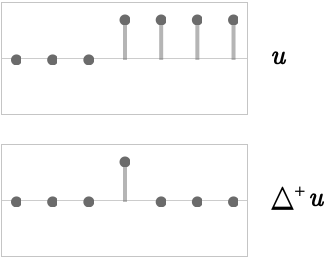Similarly we can define the backward finite difference to be $$\backwardDifference{}\,\vertexField{u} = \pathBackwardTranslate{}{\vertexField{u}} - \vertexField{u}$$, illustrated below: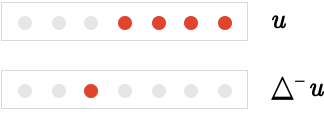The worked calculation is shown below: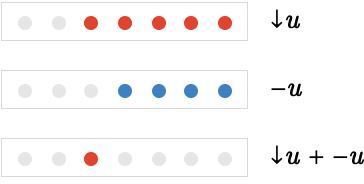We can again visualize this as a finite difference of a 1D discrete function: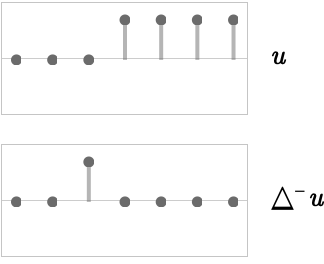Finally we can define the central difference $$\centralDifference{}\,\vertexField{u} = \forwardDifference{}\,\vertexField{u} + \backwardDifference{}\,\vertexField{u}$$, which simplifies to $$\pathBackwardTranslate{}{\vertexField{u}} - \pathTranslate{}{\vertexField{u}}$$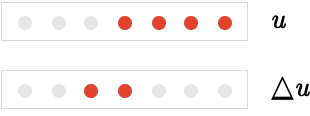The 1D discrete function interpretation: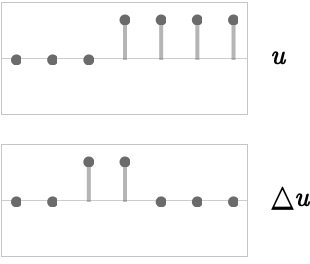### Second order differences

It is perfectly well defined to apply the $$\centralDifference{}$$ operators multiple times, which we’ll write as $$\centralDifference{}\,\centralDifference{} = \centralDifference{}_2$$, etc.

Let’s visualize the behavior of the second order forms of the three operators: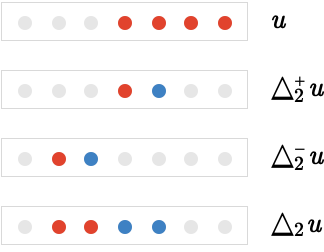### Liebniz’s rule

In traditional calculus, we have the famous Leibniz (or product) rule: $$\partialdof{\function{f} \, \function{g}} = \function{f} \, \partialdof{\function{g}} + \function{g} \, \partialdof{\function{f}}$$.

Does the same rule hold for the finite difference of a product $$\vertexField{u} \, \vertexField{w}$$ of vertices fields $$\vertexField{u}$$, $$\vertexField{w}$$ on the line lattice? The following table illustrates on the first row example vertex fields $$\vertexField{u}$$, $$\vertexField{w}$$ and their product $$\vertexField{u} \, \vertexField{w}$$, on the second row the central finite difference of them individually, and of their product, and on the third row the two terms of the ordinary product rule, and their sum.As we can see, the two bolded expressions are different, so the “naive” form of the Leibniz rule does not hold.

Luckily, it is straightforward to derive a modified form of the Leibniz rule, involving translations of one or both of the products. There are in fact two, equivalent versions of this modified form for each derivative, which one might call the ‘forward’ and ‘backward’ forms: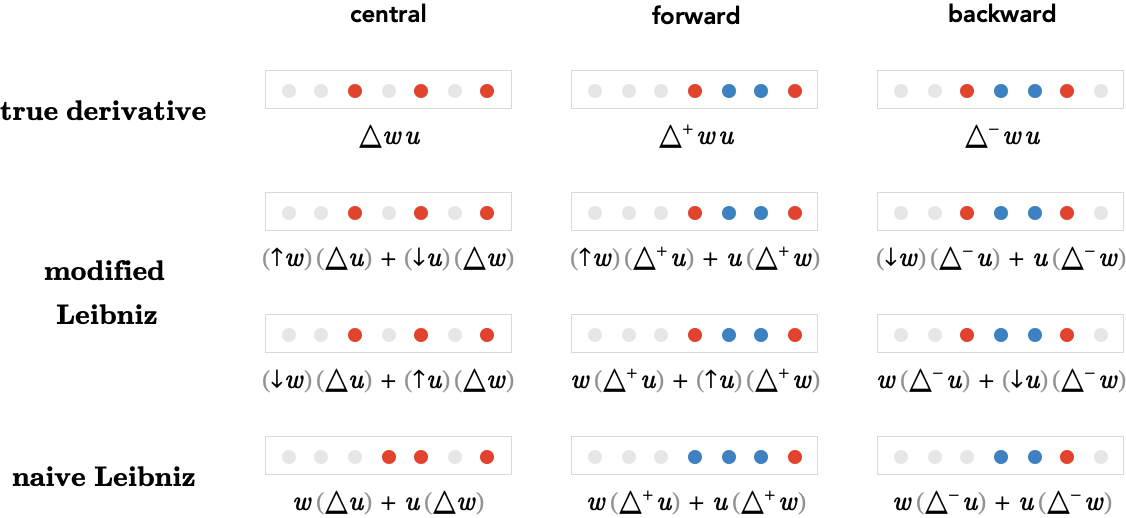Again, notice that the naive Leibniz rule does not give the true derivative for each of the three cases, whereas both the left and right forms of the modified Leibniz rule do.

It is intuitive that in the “continuum limit” of slowly changing vertex fields on very large lattices, the effect of the infinitesimal translations like $$\pathTranslate{}{\vertexField{w}}$$ can be ignored and replaced with the untranslated fields $$\vertexField{w}$$. Doing this to the above modified Liebniz rules we pass to the classical Liebnitz rule, so for example in the case of the central difference:

$\pathTranslate{}{\vertexField{w}} \, \centralDifference{}\,\vertexField{u} + \pathBackwardTranslate{}{\vertexField{u}} \, \centralDifference{}\,\vertexField{w}\approxEqualSymbol \pathBackwardTranslate{}{\vertexField{w}} \, \centralDifference{}\,\vertexField{u} + \pathTranslate{}{\vertexField{u}} \, \centralDifference{}\,\vertexField{w}\approxEqualSymbol \vertexField{w} \, \centralDifference{}\,\vertexField{u} + \vertexField{u} \, \centralDifference{}\,\vertexField{w}$

## Two dimensional lattices

To continue our recapitulation of calculus, we next move to multivariable calculus. Taking the place of continuous functions of two real variables, we will have vertex fields on lattices with two cardinals.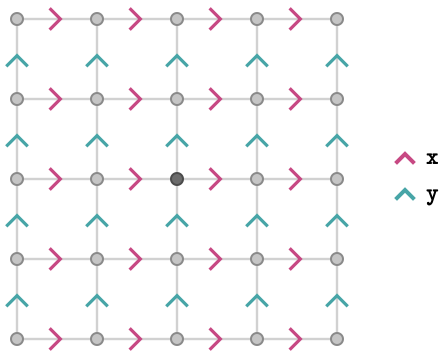This time, we will take finite difference separately in the two cardinals directions $$\rbform{\card{x}}$$ and $$\gbform{\card{y}}$$, with the cardinal writtein subscript form: e.g. for the central finite differences

zzzz
difference along $$\rbform{\card{x}}$$$$\pathCentralDifference{\card{x}}$$$$\pathForwardDifference{\card{x}}$$$$\pathBackwardDifference{\card{x}}$$
difference along $$\gbform{\card{y}}$$$$\pathCentralDifference{\card{y}}$$$$\pathForwardDifference{\card{y}}$$$$\pathBackwardDifference{\card{y}}$$

These are defined as before, except now we use per-cardinal forward and backward translations:

zzz
translation along $$\rbform{\card{x}}$$$$\pathLeftTranslate{\card{x}}$$$$\pathLeftBackwardTranslate{\card{x}}$$
translation along $$\gbform{\card{y}}$$$$\pathLeftTranslate{\card{y}}$$$$\pathLeftBackwardTranslate{\card{y}}$$

Here we show the finite differences of three simple vertex fields $$\vertexField{u}$$, $$\vertexField{v}$$, $$\vertexField{w}$$, shown on successive rows: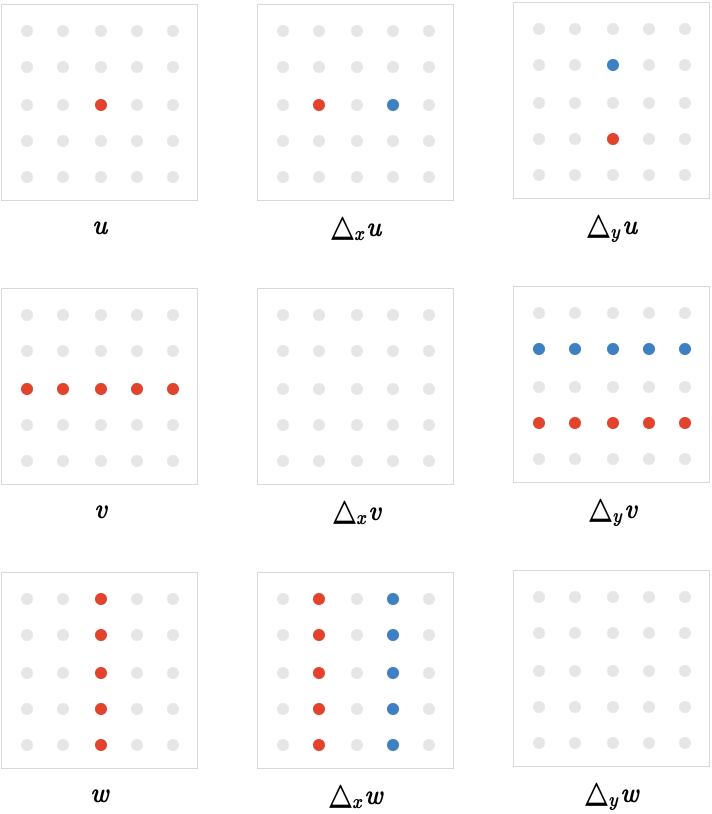We can also take second order differences in multiple ways, show here in terms of the central difference applied to $$\vertexField{u}$$. Note that we define second order differences to act in the order they appear in the subscript, so that e.g. $$\pathCentralDifference{\card{x},\card{y}} = \pathCentralDifference{\card{y}}\,\pathCentralDifference{\card{x}}$$.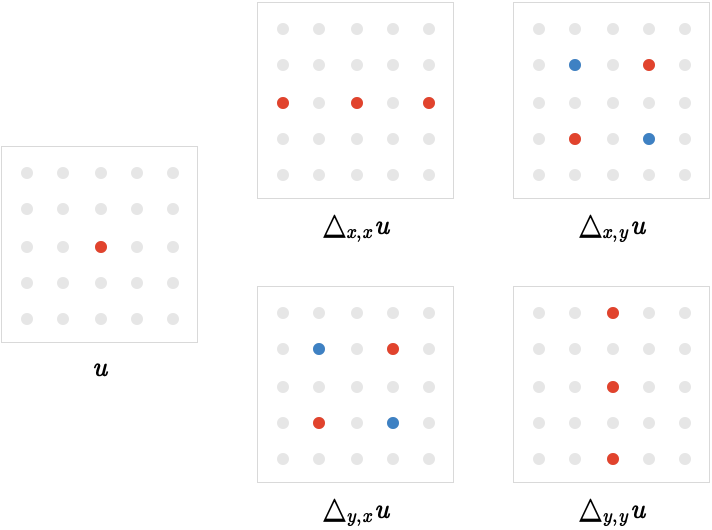Notice that $$\pathCentralDifference{\card{y},\card{x}}\,\vertexField{u} = \pathCentralDifference{\card{x},\card{y}}\,\vertexField{u}$$. In fact, this identity is true for all vertex fields. This is a consequence of the path relation $$\word{\rbform{\card{x}}}{\gbform{\card{y}}}\pathIso \word{\gbform{\card{y}}}{\rbform{\card{x}}}$$, which implies that the translations $$\pathLeftTranslate{\card{x}}$$ and $$\pathLeftTranslate{\card{y}}$$ commute, which makes the proof of the identity elementary:

\begin{aligned} \pathCentralDifference{\rbform{\card{x}},\gbform{\card{y}}}\,\vertexField{u}&= \pathBackwardTranslate{\rbform{\card{x}}}{\paren{\pathCentralDifference{\gbform{\card{y}}}\,\vertexField{u}}} - \pathTranslate{\rbform{\card{x}}}{\paren{\pathCentralDifference{\gbform{\card{y}}}\,\vertexField{u}}}\\ &= \pathBackwardTranslate{\rbform{\card{x}}}{\pathBackwardTranslate{\gbform{\card{y}}}{\vertexField{u}}} - \pathBackwardTranslate{\rbform{\card{x}}}{\pathTranslate{\gbform{\card{y}}}{\vertexField{u}}} - \pathTranslate{\rbform{\card{x}}}{\pathBackwardTranslate{\gbform{\card{y}}}{\vertexField{u}}} + \pathTranslate{\rbform{\card{x}}}{\pathTranslate{\gbform{\card{y}}}{\vertexField{u}}}\\ &= \pathBackwardTranslate{\gbform{\card{y}}}{\pathBackwardTranslate{\rbform{\card{x}}}{\vertexField{u}}} - \pathBackwardTranslate{\gbform{\card{y}}}{\pathTranslate{\rbform{\card{x}}}{\vertexField{u}}} - \pathTranslate{\gbform{\card{y}}}{\pathBackwardTranslate{\rbform{\card{x}}}{\vertexField{u}}} + \pathTranslate{\gbform{\card{y}}}{\pathTranslate{\rbform{\card{x}}}{\vertexField{u}}}\\ &= \pathBackwardTranslate{\gbform{\card{y}}}{\paren{\pathCentralDifference{\rbform{\card{x}}}\,\vertexField{u}}} - \pathTranslate{\gbform{\card{y}}}{\paren{\pathCentralDifference{\rbform{\card{x}}}\,\vertexField{u}}}&= \pathCentralDifference{\gbform{\card{y}},\rbform{\card{x}}}\,\vertexField{u}\end{aligned}

## Edge fields

Next, we are going to consider edge fields on the square lattice quiver $$\quiver{S}$$, constructed similarly to the vertex fields $$\functionSpace{\vertices{}}{\baseField{K}}$$.

An edge field on $$\quiver{S}$$ is a function $$\functionSignature{\function{\edgeField{h}}}{\edges{\quiver{S}}}{\baseField{K}}$$, where again $$\baseField{K}$$ is a field or commutative ring, and $$\edges{\quiver{S}}$$ is the set of edges of $$\quiver{S}$$. We write the space of all edge fields as $$\functionSpace{\edges{}}{\baseField{K}}$$.

#### Orientation

It's important that we consider these edges in a directed sense: an edge field is a single value associated with a given edge $$\elemOf{\edge{e}}{\edges{}}$$, namely $$\edgeField{h}(\edge{e})$$, but if we consider that edge in its invered oriention, we invert the corresponding value of the edge field: $$\edgeField{h}(\inverted{\edge{e}}) = \minus{\edgeField{h}(\edge{e})}$$. This interpretation is natural if we regard the edge field as representing a flow of some quantity, since the flow along a given edge will be negative if choose to measure it in the opposite orientation. We'll be on a firmer footing when we consider path algebra, so don't worry if this point seems vague right now.

#### Visualization

We will visualize edge fields using colored edges in the same fashion we used for vertex fields. Because they are naturally oriented by the orientation of the corresponding cardinals, a positive weight on an edge will be represented visually as a doubled-headed arrow in which the arrowhead in the forward direction is colored red, and the arrowhead in the backward direction is colored blue. The opposite applies when drawing a negative weight.

Here's an example of an edge field in which only two edges have non-zero weight: the horizontal edge has weight 1, and the vertical edge has weight -1. Alongside it is the original lattice quiver $$\quiver{S}$$ to allow comparison with the orientation of the corresponding cardinals.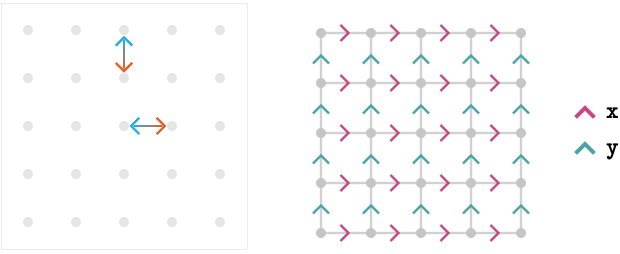We can generate an edge field from a vertex field $$\functionSignature{\function{\vertexField{u}}}{\vertices{\quiver{S}}}{\baseField{K}}$$ using the gradient operator, written $$\grad$$. Formally, $$\grad$$ is a map from the space of vertex fields to the space of edge fields, in other words $$\functionSignature{\function{\grad }}{\functionSpace{\vertices{}}{\baseField{K}}}{\functionSpace{\edges{}}{\baseField{K}}}$$. It is defined edgewise as follows:

$\function{\paren{\grad \,\vertexField{u}}}(\de{\vert{x}}{\vert{y}}) = \vertexField{u}(\vert{y}) - \vertexField{u}(\vert{x})$

In other words, the gradient operator measures the difference between the value of $$\vertexField{u}$$ at the head and tail of each edge.

Let's visualize the behavior of the gradient operator on some simple vertex fields: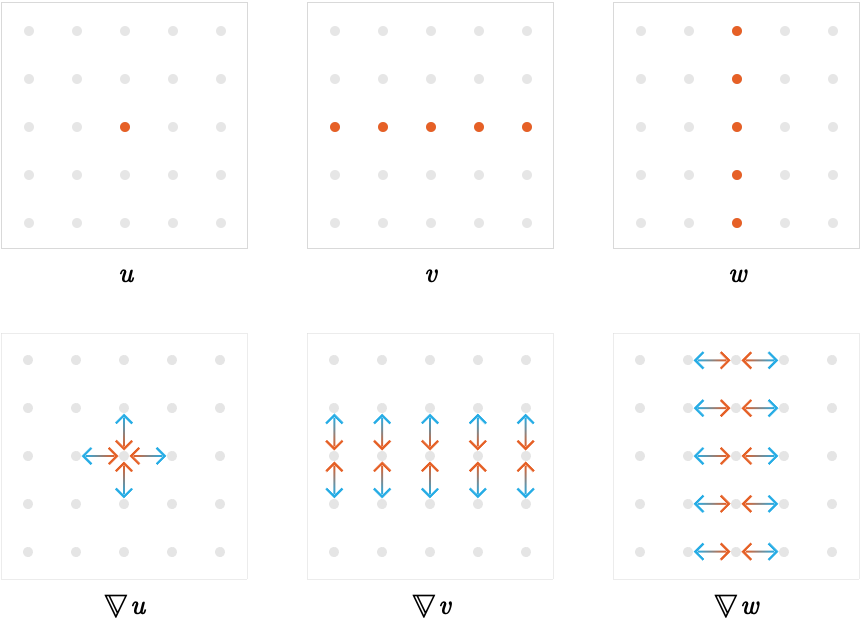### Divergence

Next we'll introduce the divergence operator, which is a kind of complementary operator to the gradient operator. The divergence operator is written $$\div$$. The divergence operator operates on an edge field $$\functionSignature{\edgeField{h}}{\edges{}}{\baseField{K}}$$ and produces a vertex field $$\functionSignature{\function{\div \,\edgeField{h}}}{\vertices{}}{\baseField{K}}$$, in othe words we have $$\functionSignature{\function{\div }}{\functionSpace{\edges{}}{\baseField{K}}}{\functionSpace{\vertices{}}{\baseField{K}}}$$.

It is defined vertexwise as follows:

$\function{\paren{\div \,\edgeField{h}}}(\vert{v}) = \indexSum{\edgeField{h}(\edge{e})}{\headVertex(\edge{e}) = \vert{v}}{} - \indexSum{\edgeField{h}(\edge{e})}{\tailVertex(\edge{e}) = \vert{v}}{}$

We can define it more elegantly using the path Kronecker, $$\functionSignature{\function{ \Xi }}{\tuple{\edges{},\vertices{}}}{\baseField{K}}$$, defined as:

$\function{ \Xi }(\edge{e},\vert{v}) = \begin{cases} 1 &\text{if } \headVertex(\edge{e}) = \vert{v}\\ -1 &\text{if } \tailVertex(\edge{e}) = \vert{v}\\ 0 &\text{otherwise} \end{cases}$

Using $$\function{ \Xi }$$ we can express $$\div$$ vertexwise as a kind of convolution:

$\function{\paren{\div \,\edgeField{h}}}(\vert{v}) = \indexSum{\function{ \Xi }(\edge{e},\vert{v}) \, \edgeField{h}(\edge{e})}{\edge{e}}{}$

Let's apply the divergence operator to some example edge fields: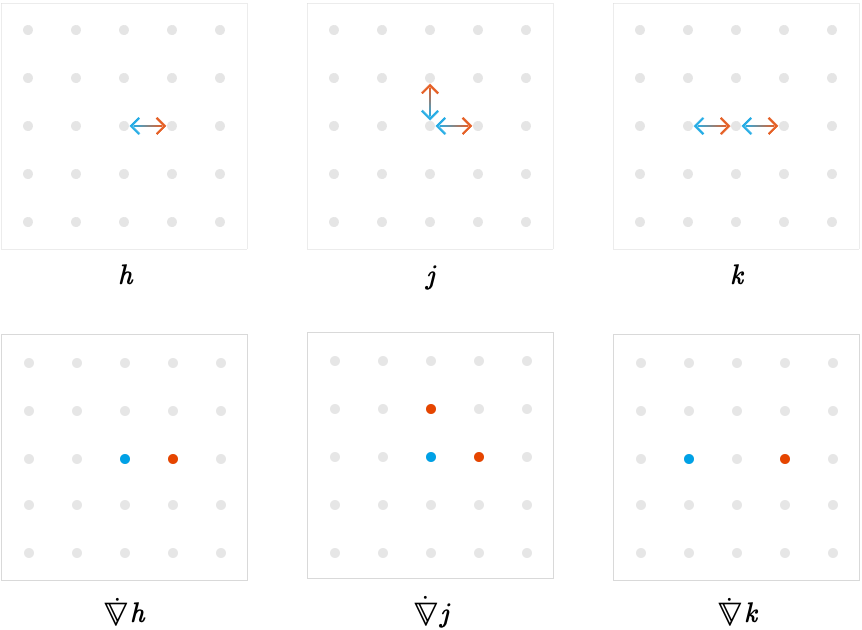### Laplacian

An interesting quantity is the divergence of the gradient, $$\laplacian = \div \,\grad$$, called the Laplacian. Let's evaluate it on a simple vertex field: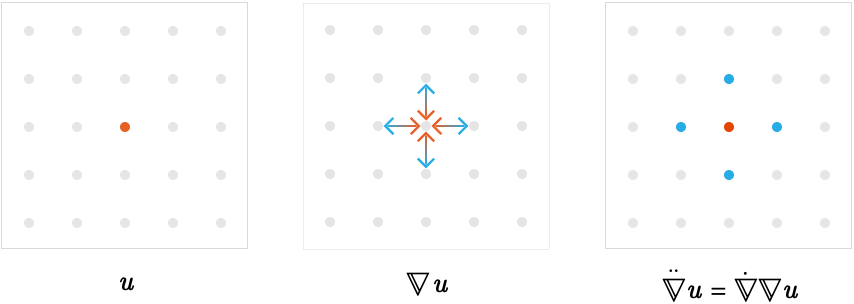The weights for vertex field $$\laplacianOf{\vertexField{u}}$$ are $$+4$$ for the central red vertex and $$-1$$ for the surrounding blue vertices. This can be explained by counting the number of incident edges for the central vertex you can see in $$\gradOf{\vertexField{u}}$$: there are 4. There is only one incident edge for the outer vertices.

We will have more to say about this at a later stage, particular in its connection with vertex colorings of lattices.

### Curl

The operator analogous to curl in vector calculus will have to wait until we have the machinery of path algebra to guide us. This is largely due to the fact that curl itself is just the tip of the iceberg, more generically handled using the idea of the exterior calculus (or, largely equivalently, geometric algebra). We will therefore leave this topic aside for now, although we'll see hints of it below.

## Integration

We've covered some basic territory in terms of finite-differential calculus. What about integration?

The staring point here is the notion of the path integral of an edge field $$\elemOf{\edgeField{h}}{\functionSpace{\edges{}}{\baseField{K}}}$$ along a path $$\path{P}$$, written $$\pathIntegral{\path{P}}{\edgeField{h}}$$.

Let's visualize this idea for an arbitrary $$\edgeField{h}$$ and $$\path{P}$$: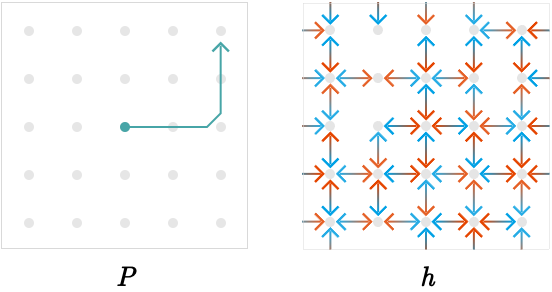We first "split" $$\path{P}$$ into its constituent edges (each edge having weight one), and then multiply them edgewise with $$\edgeField{h}$$: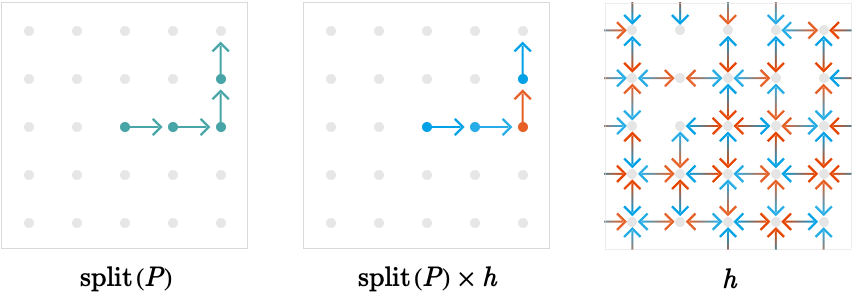The summed weight of this product vector is the result of the integral, in this case, the sum of three negative weights and one positive weight.

We can write this succintly in the following way:

$\pathIntegral{\path{P}}{\edgeField{h}} = \pathDot{\split(\path{P})}{\edgeField{h}}$

Here, the dot product or inner product between two edge fields is the map $$\functionSignature{\function{\pathDotSymbol{}}}{\tuple{\functionSpace{\edges{}}{\baseField{K}},\functionSpace{\edges{}}{\baseField{K}}}}{\baseField{K}}$$ defined to be the sum of products of their edge weights:

$\pathDot{\edgeField{g}}{\edgeField{h}} = \indexSum{\edgeField{g}(\edge{e}) \, \edgeField{h}(\edge{e})}{\edge{e}}{}$

### Fundamental theorem of discrete calculus

We can now make a rather trivial observation about the gradient edge field of a vertex field $$\vertexField{u}$$. Let's calculate the result of a path integral on $$\gradOf{\vertexField{u}}$$:

\begin{aligned} \pathIntegral{\path{P}}{\paren{\gradOf{\vertexField{u}}}}&= \pathDot{\split(\path{P})}{\paren{\gradOf{\vertexField{u}}}}\\ &= \indexSum{\suchThat{\function{\paren{\gradOf{\vertexField{u}}}}(\edge{e})}{\elemOf{\edge{e}}{\path{P}}}}{\edge{e}}{}\\ &= \indexSum{\suchThat{\vertexField{u}(\vert{y}) - \vertexField{u}(\vert{x})}{\elemOf{\de{\vert{x}}{\vert{y}}}{\path{P}}}}{\vert{x},\vert{y}}{}\end{aligned}

In other words, the integral is the sum of differences of values of $$\vertexField{u}$$ along the edges of the path $$\path{P}$$. But these will cancel in pairs, since:

$\support(\path{P}) = \list{\de{\vert{x_1}}{\vert{x_2}},\de{\vert{x_2}}{\vert{x_3}},\ellipsis ,\de{\vert{x_{\sym{n} - 1}}}{\vert{x_{\sym{n}}}}}$

And hence the integral is determined by the difference between the value of $$\vertexField{u}$$ at the head and tail of $$\path{P}$$.

$\pathIntegral{\path{P}}{\paren{\gradOf{\vertexField{u}}}} = \vertexField{u}(\headVertex(\path{P})) - \vertexField{u}(\tailVertex(\path{P}))$

This rather banal calculation amounts to a discrete version of the fundamental theorem of calculus for a function of one variable. Note the important consequence that if $$\path{P}$$ is a closed path, then by definition $$\headVertex(\path{P}) = \tailVertex(\path{P})$$, and the integral is zero.

You may have noticed that we have played a little fast and loose here: in particular, the operation $$\split$$ seemed to produce an edge field that didn't have the customary double arrowheads, and this feature was reflected by the product of it with the integrand edge field. There is good reason for this, and to understand what is going on we will have to develop path algebra. This will then allow us to formulate an appropriate discrete version of the generalized Stoke's theorem, an important milestone in "multicardinal calculus".